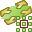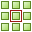# Defining a Periodic Boundary Condition (PBC)

Specify a periodic boundary condition (PBC) to analyse infinite periodic structures.

1. On the Construct tab, in the Structures group, click thePlanes/arrays icon. From the drop-down list, selectPeriodic boundaries.
2. From the Number of dimensions list, select one of the following:
• To create a one-dimensional PBC where the unit cell is repeated along a line, select One dimension.
• To create a two-dimensional PBC where the unit cell is repeated to form a surface, select Two dimensions.
• To remove the PBC from the model, select No periodic boundary.
3. Under Start point, specify the start point of the vector.
4. Under End point of first vector, specify the end point of first vector.
5. Under End point of second vector, specify the end point of the second vector.
6. Under Phase shift, select one of the following:
• When a plane wave is used as excitation, the phase difference between the cells cannot be specified. To determine the phase shift of the excitation, select Determine from plane-wave excitation.
• To specify the phase shift, select Specify manually.
• In the u1 field, specify the phase shift in the first direction, u1.
• In the u2 field, specify the phase shift in the second direction, u2.
• To specify the theta and phi angle of the “squint” angle, select Determine from beam pointing (squint) angle.
• In the Theta field, specify the theta angle of the “squint” angle.
• In the Phi field, specify the phi angle of the “squint” angle.
7. Click OK to define the PBC and to close the dialog.